###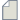Author Topic: the average Hartree difference potential under an external electric field  (Read 1325 times)

0 Members and 1 Guest are viewing this topic.

####yfchang

• Regular ATK user
•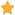• Posts: 18
• Country: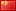• Reputation: 0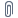##### the average Hartree difference potential under an external electric field
« on: October 24, 2019, 08:45 »
Recently, I read an article (Nano Energy, 2019, 63, 103863)，in which a methodology named OPNS (one probe and non-equilibrium surface Green’s function) was mentioned. Figure 1 herein shows that the average Hartree difference potential is perturbated by an external electric field. But in my tests, the average Hartree difference potential showed the same shape under different external electric fields. I want to know if there is something wrong with my calculation settings. I hope someone can help me.
Any help would be greatly appreciated.

####mlee

• ATK Guru
•• Posts: 173
• Country:• Reputation: 6##### Re: the average Hartree difference potential under an external electric field
« Reply #1 on: October 24, 2019, 12:00 »
You need to change the boundary condition(Right electrode: DirichletBoundaryCondition) under an external electric field as mentioned in the reference paper, while as Neumann boundary condition without an electric field in the one probe device.
When I tested it using the default condition in P-2019.03 except boundary condition(Dirichlet) and voltage(Left=0, Right=1 V to mimic the reference), I can see the HartreeDifferencePotential biased by voltage. (See the attachment)

####yfchang

• Regular ATK user
•• Posts: 18
• Country:• Reputation: 0##### Re: the average Hartree difference potential under an external electric field
« Reply #2 on: October 25, 2019, 10:33 »
Thank you so much for your help. But, why HartreeDifferencePotential at (Left=1.0, Right=0.0 V) is same as it at (Left=0.0, Right=1.0 V). Is the (Left=1.0, Right=0.0)  meaning a positive field?
PS, I used QuantumATK 2018.06-SP1-1.
« Last Edit: October 25, 2019, 10:36 by yfchang »

####yfchang

• Regular ATK user
•• Posts: 18
• Country:• Reputation: 0##### Re: the average Hartree difference potential under an external electric field
« Reply #3 on: October 28, 2019, 07:17 »
Now, by setting the right boundary condition to DirichletBoundaryCondition(vacuum + shift), the following Hartree differential potential can be obtained. Here, the vacuum is the projection of the average Hartree differential potential (about 4.4 eV) along the c-axis, and the shift is ±10 eV. the voltages of left and right electrode were set to 0 V.
My question is, is the strength of the electric field set by the shift here? If so, does the shift = 10 eV meaning that a 10 V electric field is applied to the surface? What is the relationship between the electrode voltage and the electric field?

####Petr Khomyakov

• QuantumATK Staff
• Supreme ATK Wizard
•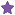• Posts: 1289
• Country:• Reputation: 25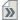##### Re: the average Hartree difference potential under an external electric field
« Reply #4 on: October 28, 2019, 16:06 »
To set a non-zero electric field for a surface configuration calculation, one may impose Neumann boundary condition with a given value for the Hartree Difference Potential, see https://docs.quantumatk.com/manual/Types/NeumannBoundaryCondition/NeumannBoundaryCondition.html. That should be done in the script. For example: 1. The electric filed E = 0.1 V/Angstrom can imposed as follows
Code
``````device_poisson_solver = FastFourier2DSolver(
boundary_conditions=[[PeriodicBoundaryCondition(),PeriodicBoundaryCondition()],
[PeriodicBoundaryCondition(),PeriodicBoundaryCondition()],
[DirichletBoundaryCondition(),NeumannBoundaryCondition(-0.1*eV/Angstrom)]],
``````
2. The electric filed E = -0.1 V/Angstrom can imposed as follows
Code
``````device_poisson_solver = FastFourier2DSolver(
boundary_conditions=[[PeriodicBoundaryCondition(),PeriodicBoundaryCondition()],
[PeriodicBoundaryCondition(),PeriodicBoundaryCondition()],
[DirichletBoundaryCondition(),NeumannBoundaryCondition(0.1*eV/Angstrom)]],
``````
3. For zero field, one may set NeumannBoundaryCondition(0.0*eV/Angstrom) or just NeumannBoundaryCondition() Note that this is not equivalent to setting the left and right electrode voltages, as there is no right electrode in the one-probe, surface configuration. One can certainly create an electric field by setting a potential value in the vacuum by using the Dirichlet boundary condition with some given potential on the right boundary, see  https://docs.quantumatk.com/manual/Types/NeumannBoundaryCondition/NeumannBoundaryCondition.html, computing the electric field in a post-processing manner from the Hartree Difference Potential in the vacuum.

####yfchang

• Regular ATK user
•• Posts: 18
• Country:• Reputation: 0##### Re: the average Hartree difference potential under an external electric field
« Reply #5 on: October 30, 2019, 03:56 »
Thank you so much for your help!